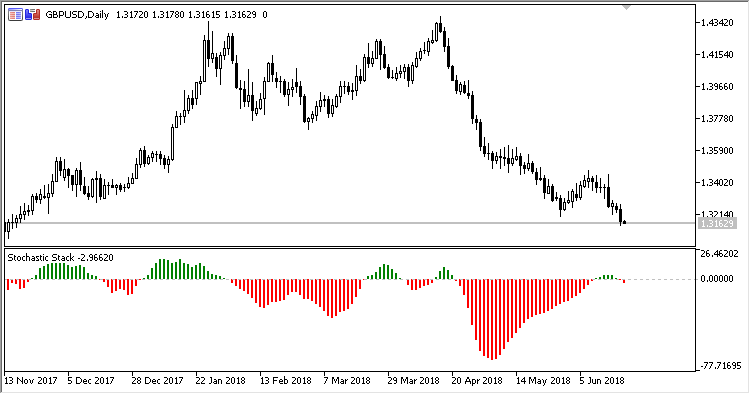# SS – indicator for MetaTrader 5

• A+
category：MT5 INDICATORS

Indicator SS (Stochastic Stack) represents a set of eight indicators Stochastic with different parameters, united in a single calculation process.

It has forty configurable parameters, five identical parameters for each of the eight stochastics:

• Stoch N %K period - period %K of stochastic N;
• Stoch N %D period - period %D of stochastic N;
• Stoch N slowing - slowing stochastic N;
• Stoch N Method - stochastic N calculation method;
• Stoch N Price field - stochastic N calculation price field.

Calculations:

`SS = (STO1 - STO2) + (STO3 - STO4) + (STO5 - STO6) + (STO7 - STO8)`